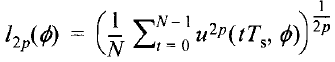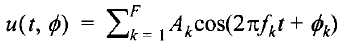# Infinity norm minimization

Hello
I have a problem. I worked with some convex problems but in this case I’m puzzled!
I would be grateful if you could help me to solve itI want to minimize the below norm using a Marquardt algorithm when I increase the p gradually (p=2,4,8,…) to find ϕk.whereAk, fk=kf0, N, fmax, F, T0 and Ts, all are known parameters.
actually t=t
Ts (sampled data)

how should I code the problem to find the ϕk?

Regards

cos is not convex, so you’re out of luck with CVX. Why isn’t CVX accepting my model? READ THIS FIRST!